Next: Summary Up: EXPERIMENTATION AT LINEAR COLLIDERS Previous: Positron Polarization

# Ground Motions

Since the vertical beam size is a few nanometers at IP for future linear colliders, the ground motion must be understood quantitatively as a function of the frequency() and the wave number(k). For this purpose, it has recently been proposed that measurements at many different places could be expressed universally by a simple formula, the so-called ATL rule.  That is, the time variance of the relative displacement(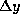) between two points can be expressed by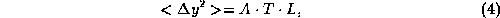where A (m/sec/m) is a coefficient which depends on various places, T(sec) is the time interval of measurements, and L(m) is the distance between the two. A simple application is the vertical ground motion between the two nearest quadrupole magnets, which are separated by 5m(=L) for JLC-I, assuming that a fast feedback can be effective at a frequency of less than 10Hz, thus T=0.1sec for the minimum time interval.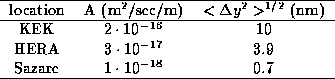Table 3: Vertical ground motions estimated using the ATL rule for various locations, assuming a distance of L=5m and a time interval of T=0.1sec.

Table 3 lists calculations for three different locations; KEK, HERA and Sazare(an old mine at a mountain-side tunnel in Shikoku island, Japan). The nanometer beams pass each other, except for Sazare, if there is no coherent movement between the two magnets. These calculations thus conclude that a rigid support system is definitely necessary.  Although this estimation is too simplified, we can clearly see how ground motion affects the final focus magnets.

M.Yoshioka (S.Takeda) presented information about the ground motions measured at four places: Sakurajima(volcanic), Sazare(green schist), ATF-KEK(solid clay) and Esashi(Granite) from south to north, in Japan, at a wide frequency region from 10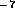to 100Hz.  Some of the results are shown in Fig.14 and compared to various locations in the world. The peaks at around 0.2Hz are due to ocean waves, while the peak at 3Hz observed at ATF-night is a resonance of Earth's crust, probably due to surrounding traffic. At ATF, the day movements were much larger than those at night at 0.110Hz, for instance 10,000 times at 1Hz. This implies that cultural noise must be seriously considered, and are dominant in real life situations. In addition, micro earthquakes have been observed at ATF, having a magnitude comparable to that of day motion. It should also be noted that there are two clusters in the power spectra at a frequency of >10Hz, where the motion of surface ground(ATF,Sazare,SLC and CERN-TT2A) is larger by an order of magnitude than those deep underground(LEP and UNK tunnels).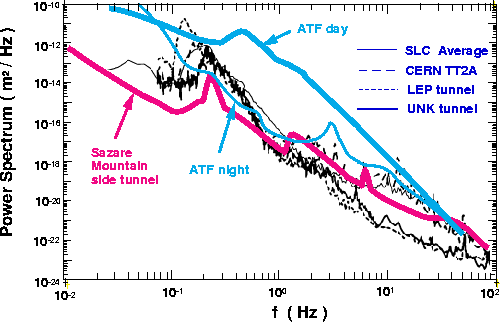Figure 14: Power-spectrum density of vertical ground motion at various locations.

C.Adolphsen had a very impressive talk concerning ground-motion tolerances based on measurements in the SLC linac tunnel by building a ground motion model.  It has been emphasized that the stability of nanometer beam collisions can be accurately estimated if we know the two dimensional(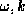) power spectrum of the ground motion at the site of the linear collider. Vertical ground motions have been simultaneously measured at 9 points located at 0, 2, 24, 50, 100,200, 400, 988, 2000m in the SLC tunnel. The data were recorded for 1.8 hours, effectively scanning at 128Hz. After a Fourier analysis, all of the data were well fitted by a model of a two-dimensional plane wave propagating on the surface of the ground with the phase velocities depending on the frequencies. Convoluting with the optics in two linacs, they first calculated the r.m.s. beam separation(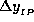) due to the ground motion in the linacs at IP, and then its Fourier components. Finally, they estimated the ground-motion tolerances as a function of the frequency (Fig.15). Compared to the tolerance with the feedback of the SLC type, which is apparently effective at a frequency of <3 Hz, the effect of ground motion turns out to be tolerable in the SLC tunnel.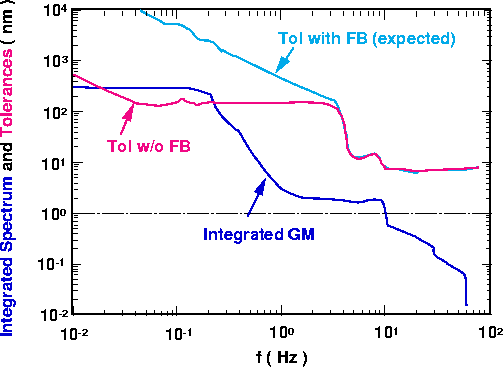Figure 15: Ground-motion tolerances and integrated spectrum as a function of the frequency, based on SLC linac measurements during ``quiet" conditions.Next: Summary Up: EXPERIMENTATION AT LINEAR COLLIDERS Previous: Positron Polarization

Toshiaki Tauchi
Fri, Dec 20, 1996 02:24:05 PM